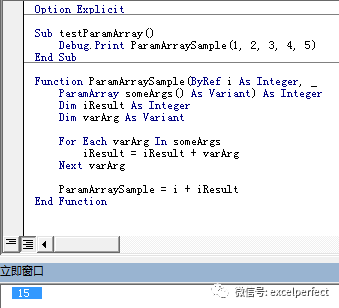# vba 自定义function返回值_Excel VBA解读（131）： 理解Function过程的使用细节

excelperfect

Function过程能够让我们自定义可以返回值的函数，减少复杂性，扩展功能，提高效率。在使用VBA编写自定义函数时，了解一些注意事项，掌握一些技术技巧，能够帮助我们顺利创造自已的函数。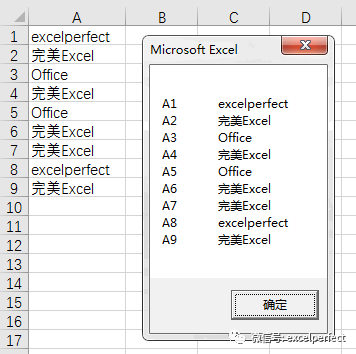Function PopulateArray(str AsString) As String()

Dim strTempArray(1 To 9) As String

Dim i As Integer

For i = 1 To 9

strTempArray(i) = str & CStr(i)

Next i

PopulateArray = strTempArray

End Function

PopulateArray函数接受所传递的字符串值，并将其与数字合并成一个字符串，然后将合并的字符串值赋值给数组元素，最后将得到的数组赋值给函数名，作为函数过程的返回值。

Sub test()

Dim i As Integer

Dim strUnion As String

Dim strArray() As String

strArray = PopulateArray("A")

For i = 1 To UBound(strArray)

strUnion = strUnion & strArray(i) _

& vbTab &Range("A" & i).Value & vbNewLine

Next i

MsgBox strUnion

End Sub

• 自定义函数的返回值可以是指定数据类型的数组，本例中为String型数组。

• 要使自定义函数的返回值是指定数据类型的数组，必须在自定义函数声明时，在数据类型后添加括号。

• 在调用过程中，接受自定义函数所返回数组的数组应声明为动态数组，其大小未显式定义。

• 在调用过程中，调用自定义函数所返回的数组必须赋值给相同类型的数组。

Sub testVarArray()

Dim i As Integer

Dim myArray As Variant

myArray = varArray()

For i = LBound(myArray, 1) To UBound(myArray, 1)

Debug.Print myArray(i, 1)

Next i

End Sub

Function varArray() As Variant

varArray = Range("A1:A9")

End Function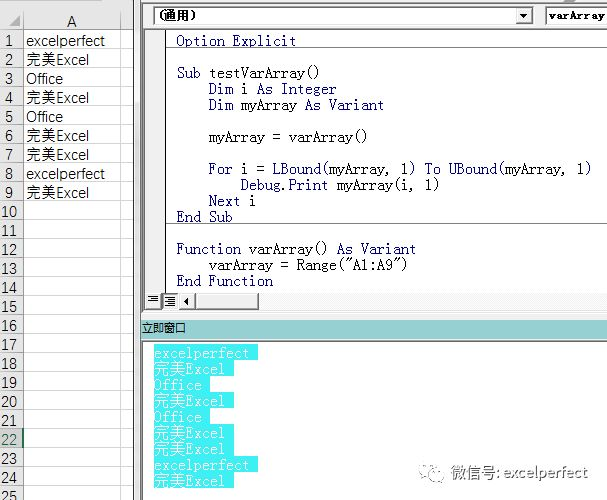Sub testReturnValue()

Dim i As Integer

i = 1

If ReturnValue(i) = True Then

Debug.Print i

End If

End Sub

Function ReturnValue(ByRef myValueAs Integer) As Boolean

myValue = myValue + 1

ReturnValue = True

End Function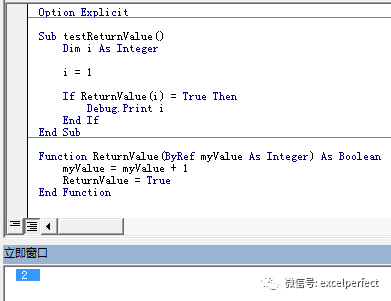Sub testOptionArgument()

Dim i As Integer

Dim iOption As Integer

i = 1

iOption = 100

Debug.Print OptionArgument(i)

End Sub

Function OptionArgument(ByRef iValAs Integer, _

Optional iTwo As Integer)

If IsMissing(iTwo) Then

OptionArgument = iVal

Else

OptionArgument = iVal + iTwo

End If

End Function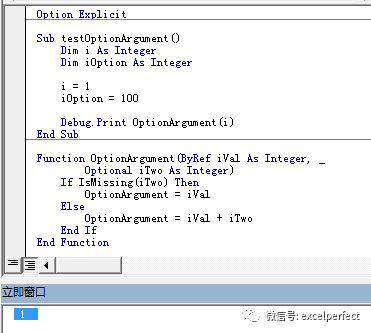• 必须为Variant类型的数组。

• 必须在声明的最后部分。

Sub testParamArray()

Debug.Print ParamArraySample(1, 2, 3, 4, 5)

End Sub

Function ParamArraySample(ByRef iAs Integer, _

ParamArray someArgs() As Variant) As Integer

Dim iResult As Integer

Dim varArg As Variant

For Each varArg In someArgs

iResult = iResult + varArg

Next varArg

ParamArraySample = i + iResult

End Function